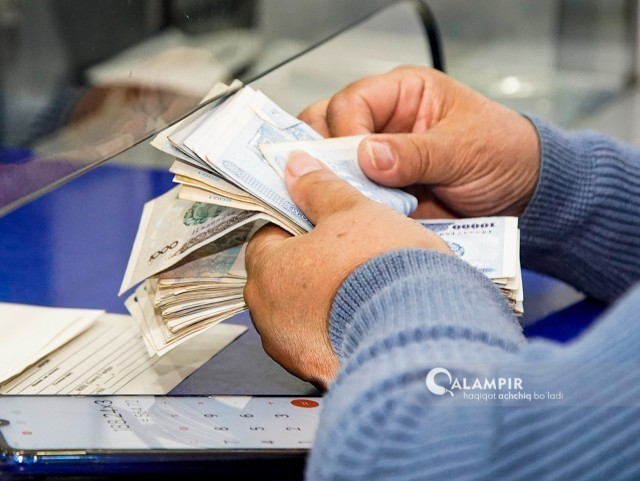# Uzbekistan’s cash in circulation has decreased by almost half a trillion

LocalFollowing the devaluation of the soum, the cash in circulation in Uzbekistan decreased by 445 billion sums to 46.6 trillion sums.  This is mentioned in the information of the central bank.

As of August 1, the money supply in the national currency (sum) has decreased by almost 1 trillion sums and is 148.1 trillion sums.  This situation was also observed at the beginning of the year, when the money supply in the national currency decreased by 4.5 trillion sums in one month.

In addition, in July, deposits in soums fell to 39.9 trillion sums.  This means that the amount has decreased by 1.7 trillion sums compared to the previous period.

However, due to the devaluation of the soum, dollar deposits increased for the first time in 8 months.  At the same time, Uzbekistan’s foreign currency deposits have reached 44.9 trillion sums.  However, this is not due to the increase of dollars in the country, but to the fall of the soum by 400 sums in July, that is, to its devaluation.

In short, the dollar increased where it stood, and the sum did the opposite.